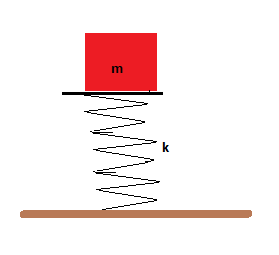# A spring oriented vertically is attached to a hard horizontal surface as in the figure below. The...

## Question:

A spring oriented vertically is attached to a hard horizontal surface as in the figure below. The spring has a force constant of 1.46 kN/m. How much is the spring compressed when a object of mass m = 2.30 kg is placed on top of the spring and the system is at rest?## Hooke's Law:

Let us assume a flexible plastic having force constant (k) is compressed by a distance (x) by applying an external force of magnitude (F). In this example, the flexible plastic rod will impose a force of similar magnitude in the reverse direction. In this case, the force (F) will be directly proportional to compression (x) caused in the plastic rod.

## Answer and Explanation: 1

Become a Study.com member to unlock this answer! Create your account

Given data:

• Mass of the object, {eq}m = 2.30 \ kg {/eq}
• Spring constant, {eq}k = 1.46 \ k N/m {/eq}

Let the compression in the spring be x.

From...

See full answer below.

#### Learn more about this topic:Hooke's Law & the Spring Constant: Definition & Equation

from

Chapter 4 / Lesson 19
202K

After watching this video, you will be able to explain what Hooke's Law is and use the equation for Hooke's Law to solve problems. A short quiz will follow.